### Exercise 1.6 from Stein and Shakarchi’s Fourier Analysis

The problem is as follows:

We have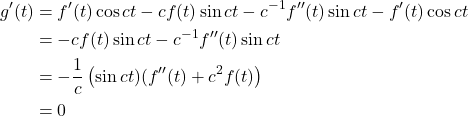and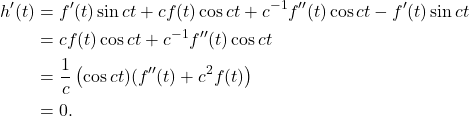Each equality with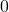follows from the assumption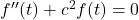. Therefore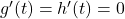and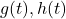are constant.

The problem asks for constants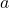and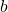. Could it be that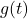and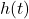are these constants? That would be a natural guess. Let’s just try computing the sum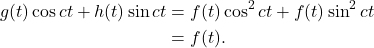.

So indeed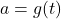and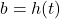.

May 10th, 2023 | Posted in Math